## Indian Journal of Agricultural Research

• Chief EditorT. Mohapatra

• Print ISSN 0367-8245

• Online ISSN 0976-058X

• NAAS Rating 5.20

• SJR 0.293

Frequency :
Bi-monthly (February, April, June, August, October and December)
Indexing Services :
BIOSIS Preview, ISI Citation Index, Biological Abstracts, Elsevier (Scopus and Embase), AGRICOLA, Google Scholar, CrossRef, CAB Abstracting Journals, Chemical Abstracts, Indian Science Abstracts, EBSCO Indexing Services, Index Copernicus
Indian Journal of Agricultural Research, volume 56 issue 1 (february 2022) : 47-56

# ​Rainfall Probability Distribution and Forecasting Monthly Rainfall of Navsari using ARIMA Model

D.K. Dwivedi1,*, P.K. Shrivastava1
1Department of Natural Resource Management, College of Forestry, ACHF, Navsari Agricultural University, Navsari-396 450, Gujarat, India.
• Submitted17-04-2021|

• Accepted12-07-2021|

• First Online 31-07-2021|

Cite article:- Dwivedi D.K., Shrivastava P.K. (2022). ​Rainfall Probability Distribution and Forecasting Monthly Rainfall of Navsari using ARIMA Model . Indian Journal of Agricultural Research. 56(1): 47-56. doi: 10.18805/IJARe.A-5793.
Background: Reliable rainfall forecast could be helpful to farmers as major decisions regarding selection of crops and sowing time are based on the rainfall. The univariate time series ARIMA model requires only past data for model formulation and to simulate stochastic processes. The current study was aimed to obtain the probability distribution of monthly rainfall using the method of moments and to forecast rainfall using the ARIMA model.

Methods: The method of moments was used to determine the parameters of distributions and the chi-square test was used as a goodness of fit test to obtain the best fit distribution for monthly rainfall of Navsari, Gujarat utilizing 36 years of rainfall data. Auto regressive moving average (ARIMA) model, popular owing to its simplicity and ability to simulate various stochastic processes was used in the study.

Result: It was revealed that the Weibull distribution was the best fit distribution for June and September, whereas Gumbel was the best fit distribution for July. For simulating monthly rainfall, the seasonal ARIMA model (0,0,1) (0,1,1)12 was found to be the appropriate model based on its performance. The model had the least root mean square value and also the residuals were found to have no correlation.
The pattern of rainfall and its magnitude is important for agriculture and water resource management. For stochastic events such as rainfall, continuous probability distributions are generally used as the set of possible outcomes have values in a continuous range. The continuous probability distributions can be described by probability density function and cumulative density function (Erhan, 2011).  In this study, the probability density functions of various continuous probability distributions like normal, log-normal, exponential, gamma, weibull, gumbel and generalized extreme value which are generally applicable in stochastic hydrology were used.

The Smirnov anderson and Chi-square tests were used by Sharma and Singh (2010) to obtain the best fitting probability distribution for monthly, seasonal and annual rainfall of Pantnagar and it was found that the lognormal and gamma distribution were the best fit probability distributions for the annual and monsoon season period of study, respectively. The goodness of fits namely Smirnov and Anderson were utilized by Mandal and Choudhury (2015) to obtain the probability distribution of  Sagar Island, located on the continental shelf of the Bay of Bengal and found that Normal distributions were appropriate for annual, post-monsoon and summer seasons. Trend analysis helps in assessing the positive or negative trends of rainfall. Brema and Anie (2018) analysed the rainfall trend by Mann Kendall test for Vamanapuram river basin, Kerala by using 30 years (1984-2013) of rainfall data. For January, May, June, September, October and November there was an evidence of rising trend while negative trend was observed in February, March, April, July, August and December.

Reliable rainfall forecast could be a boon to farmers as many important decisions about sowing time and selection of crops are based on the rainfall. Auto regressive moving average (ARIMA) is a linear model, popular owing to its simplicity and ability to simulate various stochastic processes (Adhikari and Agrawal, 2013).  The ARIMA model was utilized by Swain et al., (2018) for predicting monthly rainfall over Khodha district in Odisha and concluded that ARIMA (1, 2, 1) (1, 0, 1)12 was the best fit model.

This study was undertaken with the objectives of obtaining the best fit distribution of monthly rainfall (1984-2019) of Navsari from a set of continuous probability distributions using chi-square as the goodness of fit test and to develop best fit ARIMA model for monthly rainfall of Navsari.
The rainfall data of 36  years (1984-2019) of Navsari, Gujarat was analysed to obtain the best fit monthly and annual probability distribution. The analysis required in the study was conducted in the year 2020. Navsari receives its rainfall during June, July, August and September. Water conservation measures can be planned based on the expected rainfall.  The excessive rainfall also causes frequent inundation and therefore, suitable drainage can also be planned for protecting the crops. The continuous probability distributions with their probability density functions used in the study are described below.

Normal distribution

The normal distribution, also known as Gaussian distribution is one of the most frequently used distributions to model the random phenomenon. Any linear function of a random variable is also a normal random variable. The probability density function of normal distribution is given by equation (1):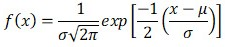...........(1)
for -∞< x < ∞, -∞< µ < ∞ and 𝛔 > 0

μ and σ are the mean and standard deviation of the distribution which are also its location and scale parameters. The parameters of the distribution were determined using method of moments in which the mean and the standard deviations were obtained.

Log normal distribution

Log-normal distribution is a transformed normal distribution where the variable is replaced by its logarithmic value. It has positive skewness which increases with its scale parameter. A random variable x is log-normally distributed if its probability density function is as shown by equation (2).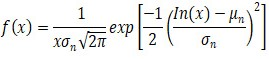..........(2)
for -∞< x < ∞, -∞< mn < ∞ and 𝛔n > 0,

In which mn and sn are scale and shape parameters of the distribution respectively.

The scale and shape parameters are also the mean and variance of the variable ln x. The two parameters of this distribution can be obtained using method of moments using equations (3) and (4).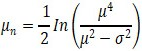..........(3)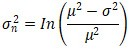..........(4)

Gamma distribution

Gamma distribution is a flexible distribution with a wide variety of shapes. A random variable x follows gamma distribution if its probability density function is given by equation (5):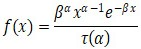..........(5)

In which a and b are shape and scale parameter of distribution respectively.

The method of moments was used to estimate the parameters of the distribution as given in equation (6).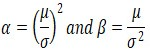...........(6)
Where
µ and 𝛔 are the mean and standard deviation of the distribution respectively.

Gumbel distribution

It is the extreme value type I distribution where the parent distribution is unbounded in the direction of the desired extreme and all the moments of the distribution exist. The probability distribution function for this distribution is given by equation (7).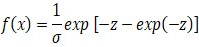...........(7)
for -∞< x < ∞, where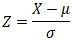𝛔 is the scale parameter and µ location parameter of the distribution.

Weibull distribution

It is the extreme value type III distribution in which the parent distribution is bounded in the direction of the desired extreme. The probability distribution function for this distribution is given by equation (8).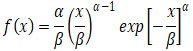...........(8)
for 0 ≤ x < ∞, α, β > 0

α is the scale parameter and b is location parameter of the distribution.

The mean and variance are given by the following equations (9) and (10).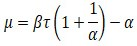...........(9)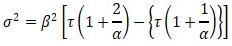...........(10)

Goodness-of-fit test

The chi-square test was used for checking the validity of the assumed probability distribution. If more than one distribution passed the test then the distribution with the least value of chi-square was considered as the best fit distribution (Greenwood and Nikulin, 1996). The chi-square statistic is given by equation (11).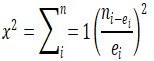..........(11)

Where,
ni = Observed value.
ei = Expected value.

Mann kendall test

This test is used for the purpose of statistically assessing if there is a monotonic upward or downward trend of the variable of interest over time (Mann, 1945; Kendall, 1975). According to this test, the null hypothesis H0 assumes that there is no trend (the data is independent and randomly ordered) and this is tested against the alternative hypothesis H1, which assumes that there is a trend.

Auto regressive integrated moving average (ARIMA) model

The formulation of ARIMA model required three steps, namely model identification, parameter estimation and diagnostic checking for analysis of residuals (Box and Jenkins, 1976). The ACF and PACF plots were used for identifying the order for the autoregressive and moving average terms.

The seasonal ARIMA model is given as follows:

ΦP (Bs) φp (B) sD d zt = θq (B)ΘQ(Bs)at        ..........(12)

ΦP (Bs) = Seasonal autoregressive operator of order P.
φp = Regular autoregressive operator of order p.
sD = Seasonal differences.
d = Regular differences.
ΘQ (Bs) = Seasonal moving average operator of order P.
θq (B)= Regular moving average operator of order p.
at= White noise process.

Ljung-Box test was used for testing the residuals. This statistic measured the significance of residual autocorrelations as a set and pointed out if they were collectively significant (Paretkar, 2008).
The descriptive statistics of the monthly rainfall (Table 1), the best fit probability distributions of the monthly and annual rainfall (Table 2) and the best fit distribution along with the corresponding cumulative distribution plot and P-P plot (Fig 1) of Navsari is presented. The monthly rainfall and annual rainfall at various probabilities (Table 3), recurrence intervals  (Table 4) and the scatter plots of rainfall (Fig 2) and probability (Fig 3) is similarly presented. The rainfall values corresponding to various recurrence intervals for monthly and annual rainfall are shown in Fig 4 and Fig 5. The trend line equations based on rainfall and probability plot are given in Table 5. The study conducted by Bhakhar et al., (2008) revealed that Gumbel distribution was the best fit distribution for monthly rainfall in Kota. In the present study, Gumbel distribution was found to be the best fit distribution characterising the rainfall in the month of July.

The highest average rainfall (647.3 mm) occurred in July followed by August (344 mm) and June (317.8). It was lowest (264.04 mm) in the month of September compared to the other months included in monsoon season. The percentage of contribution to average annual rainfall was 19.6% (June), 39.9% (July), 21.2% (August) and 16.3% (September). Thus, the four months of monsoon contributed a total of 97.1% of average annual rainfall and the remaining months contribute only 2.9%. Weibull distribution was found to be the best fit distribution for June and September whereas for July and August month Gumbel and log normal distributions were found to be the best fit distribution. The design of temporary as well as permanent structures is based on the rainfall at various recurrence intervals. Usually, the recurrence interval of annual rainfall is taken into consideration for designing of structures and planning of watersheds. The results obtained about rainfall at various recurrence intervals in this study can be used by policy decision and planning related to soil and water conservation as well as crop planning.

The result of the trend analysis by Mann Kendall test for monthly and annual rainfall is given in Table 6. The time series plots of the monthly and annual rainfall showing increasing or decreasing trend is shown in Fig 6 and Fig 7 respectively. In this study, the trend was found to be significant for rainfall of September month as indicated by the standardized S statistic value (Z value) of 2.342 which was greater than the critical value of 1.645 at 5% significance level. There was insufficient evidence to suggest a significant trend in case of June, July, August and annual rainfall. The trend for annual rainfall was positive as suggested by the positive value of Mann Kendall test value (S), however, the trend was insignificant.

Auto regressive moving average (ARIMA) model

The preliminary examination of autocorrelation function (ACF) plot and partial autocorrelation (PACF) function plot revealed the presence of periodicities indicating a non- stationary process which was subsequently transformed into a stationary process by differencing. The monthly rainfall data series was converted into a stationary time series (Fig 8 and Fig 9). The performance of the ARIMA model was assessed using 5 years monthly data (2015-2019). Root Mean square errors of several candidate models were calculated and the model with least mean square error was chosen for modelling monthly rainfall. Karthika et al., (2017) utilized ARIMA model to forecast meteorological drought up to 2 years in lower Thirumanimuthar sub-basin in Tamil Nadu and the predicted data show reasonably good agreement with the actual data.

In the present study, ARIMA (0,0,1) (0,1,1)12 was selected as the appropriate model as the performance on the testing data was better compared to other candidate models and the residuals were found to have no correlation.  The data in Table 7 shows the parameters of the selected ARIMA model while that in Table 8 depicts the performance of the ARIMA model in terms of root mean square error for the training and testing period. The observed and predicted rainfall during training and testing period by ARIMA (0,0,1) (0,1,1) 12 are as in Fig 10 and Fig 11. The Ljung box values and residuals plots are shown in Fig 12. The residuals lay within bounded limits which meant that the residuals were uncorrelated and they followed white noise. The selected model was used for predicting monthly rainfall of the year 2020 as shown in Fig 13.  The model predicted that in the year 2020, the rainfall in the monsoon months i.e. June, July, August and September would be 339 mm, 680 mm, 426 mm and 349 mm respectively and the total annual rainfall would be 2062 mm which is 27.2% more than the average annual rainfall of Navsari.

The study was undertaken to ascertain the probabilities of monthly and annual rainfall using the best fit probability distribution. It was concluded that for Navsari, the Weibull distribution was found to be the best fit distribution for June and September while Gumbel and log normal distribution were found to be the best fit distribution for July and August month, while Gumbel distribution is the best for annual rainfall. The rainfall at various probabilities and recurrence interval were obtained which could be useful for design of water conservation structures as well as for structures used for checking erosions. The trend analysis by Mann kendall test suggested that for Navsari, the trend was significantly negative for June rainfall while it was positive for July and August months. Seasonal ARIMA (0,0,1)(0,1,1)12 was chosen as the appropriate model for predicting monthly rainfall of Navsari as the residuals were found to be uncorrelated and it had the least root mean square value compared to other ARIMA models with different parameters.
The authors are thankful to the Department of Agro-meteorology, Navsari Agricultural University for providing the data required for the research work. The authors are also thankful to the Department of Science and Technology, Government of India, New Delhi for facilitating the research study.

View all (0)